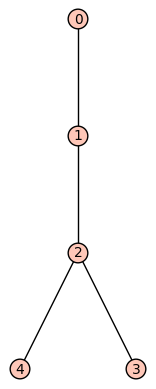# Tree layout of a graph

Consider the graph of this tree:

T = Graph()
E = [(0, 1), (1, 2), (2, 3), (2, 4)]
T.show(layout='tree',tree_root=0,tree_orientation='down')I would like to see the edges displayed in the order as given in the list E from left to right. In other words, I would like to have node(4) exchange its position with node(3).

How can I achieve this?

Edit: To clarify: Changing the order of the edge-list is no option (besides not working either). The order of the edges must be adhered to the order given.

edit retag close merge delete

Sort by » oldest newest most votedI tried with success changing the order of edges in E and converting to strings.

T = Graph()
E = [(0, 1), (1, 2), (2, 4), (2, 3)]
E = [map(str,e) for e in E]
T.show(layout='tree',tree_root='0',tree_orientation='down')

more

I do not want to change the order of the edges. This is a minimal example; in reality the edges are a large and complicated sequence computed by a program. I want to persuade Sage to respect the order of the edges as given.You can use instead planar graphs by setting the embedding:

sage: T = Graph()
sage: E = [(0, 1), (1, 2), (2, 3), (2, 4)]
sage: T.set_embedding({0:,1:[0,2],2:[1,3,4],3:,4:})
sage: T.show()

more

This might work; however the 'set_embedding' requires additional work with the edges and is not satisfying as it is completely unnecessary since the requested embedding is already there: implicitly in the list of the edges. All I want is that Sage respects the given order instead of messing it up. Thus my question seems to amount to a quite natural feature request.